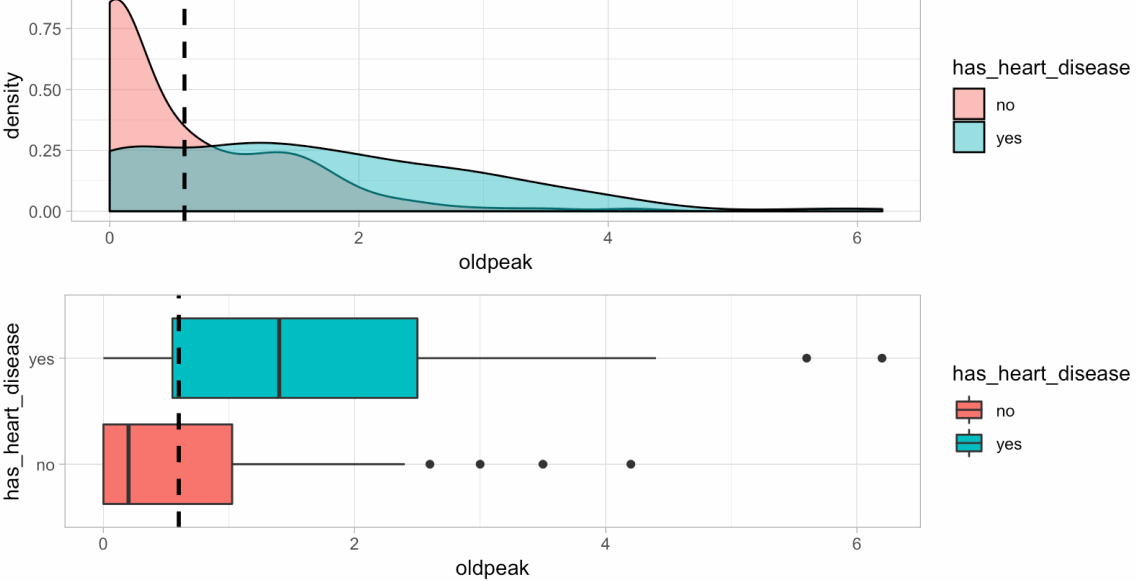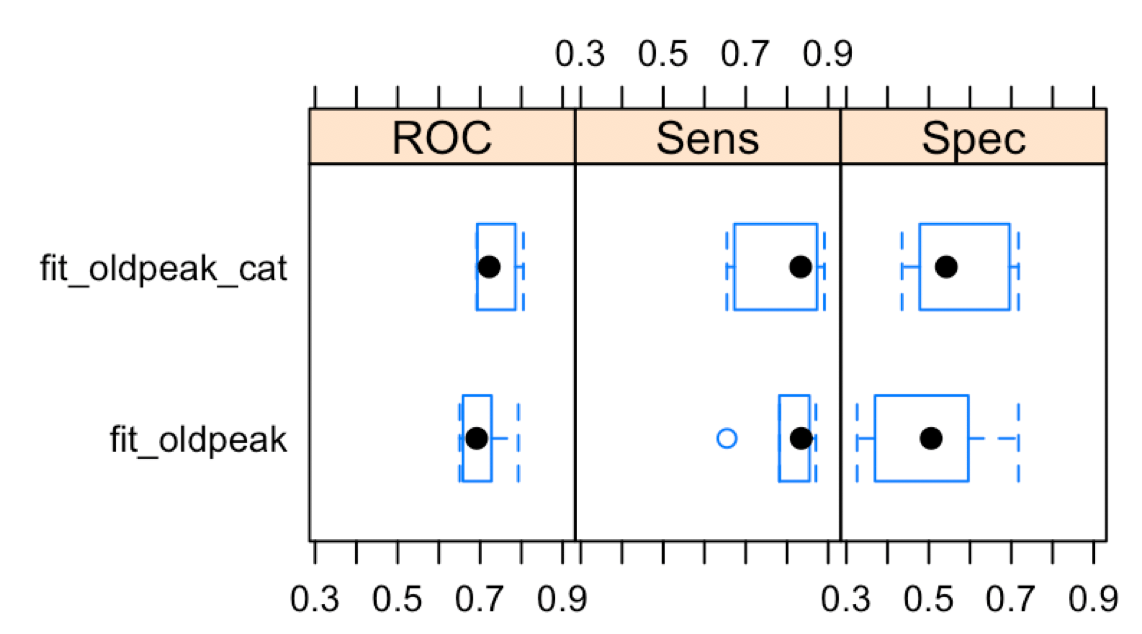[This article was first published on R - Data Science Heroes Blog, and kindly contributed to R-bloggers]. (You can report issue about the content on this page here)
Want to share your content on R-bloggers? click here if you have a blog, or here if you don't.Hello everyone, I’m happy to share a new method to discretize variables I was working on for the last few months:

Recursive discretization using gain ratio for multi-class variable

tl;dr: `funModeling::discretize_rgr(input, target)`

The problem: Need to convert a numeric variable into one categorical, considering the relationship with the target variable.

How do we choose the split points for each segment? The selection can improve or worsen the relationship.

## Example

```# Available from version 1.7 (2019-02-13), please update it before proceeding:
# install.packages("funModeling")
library(funModeling)
library(dplyr)

heart_disease\$oldpeak_2 = discretize_rgr(input=heart_disease\$oldpeak, target=heart_disease\$has_heart_disease)
```

Check the results:

Before and after the transformation

```head(select(heart_disease, oldpeak, oldpeak_2))

##   oldpeak oldpeak_2
## 1     2.3 [1.9,6.2]
## 2     1.5 [1.4,1.9)
## 3     2.6 [1.9,6.2]
## 4     3.5 [1.9,6.2]
## 5     1.4 [1.4,1.9)
## 6     0.8 [0.6,1.0)
```

Checking the distribution

```summary(heart_disease\$oldpeak_2)

## [0.0,0.6) [0.6,1.0) [1.0,1.4) [1.4,1.9) [1.9,6.2]
##       135        31        34        39        64
```

Plotting

```cross_plot(heart_disease, input = "oldpeak_2", target = "has_heart_disease")
```Left: accuracy, right: representativeness (sample size).

More info about `cross_plot` here.

## Parameters

• `min_perc_bins`: Controls the minimum sample size per bin, `0.1` or 10% as default.
• `max_n_bins`: Maximum number of bins to split the input variable, `5` bins as default.

Both parameters are related, in the sense that setting a higher number in `min_perc_bins` may not satisfy the number of desired bins (`max_n_bins`).

## Little benchmark

Next image shows ROC metrics for two models, one with the original variable and another with the discretized variable. In this case, the discretization improves ROC value, but decreases the specificity.## Other scenarios

### Case 1: Missing values in numeric variables.

In this case the way we discretize a variable weight more heavily. One data preparation trick is to convert it to categorical, when one category is `"NA"` and the remaining categories are the bins calculated by the algorithm. `funModeling` supports this scenario for equal frequency discretization, and will do the same for `discretize_rgr`.

### Case 2: Exploratory data analysis

From the discretization, we can semantically describe the relationship between the input and the target variable. Finding the segments that maximizes the likelihood might be quite helpful to report in our job or research.

• It keeps a minimum sample size per segment (representativity), thanks to `min_perc_bins`
• It uses the gain ratio metric to calculate the best split point that maximizes the target variable likelihood (accuracy).

The control of minimum sample size helps to avoid bias in segments with low representativity.

Gain ratio is an improvement over information gain, commonly used in decision trees, since it penalizes variables with high cardinality (like zip code).

The method find the best cut point based on a list of possible candidates. Each candidate is calculated based on the percentiles. Once it finds a point that maximizes gain ratio while at the same time, satisfy the condition of minimum sample size, it creates two search branches considering all the rows above and below the cutpoint, the left and the right respectevelly.

Now again, for each branch the algorithm finds the best point, for that subset of rows, and the process repeats recursivelly until satisfy the stopping criteria.

The Data Science Live Book covers some points related to this method:

Want to grasp more about the information theory world? A Simple Guide to Entropy-Based Discretization by Kevin Meurer.

Leave in the comments any doubt 😉